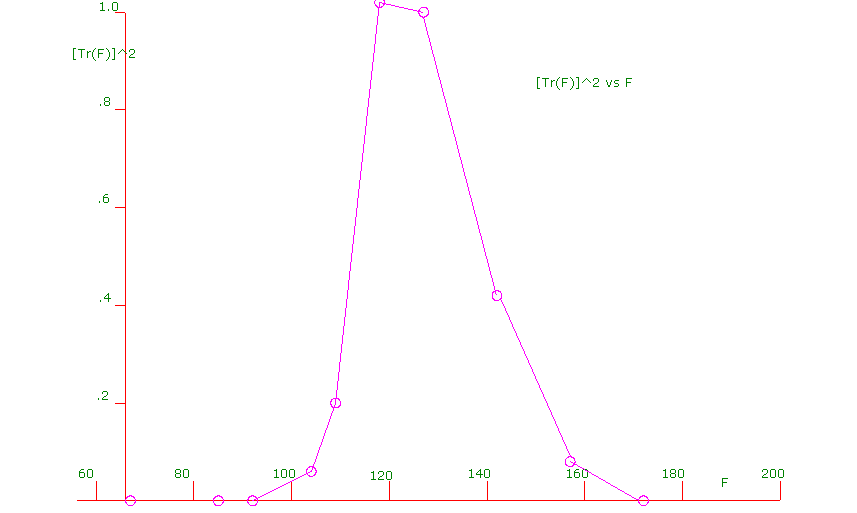# XYLENE POWER LTD.

## POWER LINE CARRIER LIGHTING CONTROL

#### SYSTEL DATA FOR [Tr(F)]^2 AS A FUNCTION OF FREQUENCY F FOR PLC RECEIVERF(kHz)---Tr(F)---[Tr(F)]^2--- Area
67.01274.0001620
85.01380.0001900
92.06684.0044680
104.2540.064516.414
109.4466.199452.660
1181.01161.0233355.502
1271.01.09.105
142.6456.41679910.626
157.2917.0850893.764
172.0490.002401.656

The Integral{[Tm(F)]^2}dF is simply the area under the above curve.

Integral{[Tr(F)]^2}dF = 30.727 kHz

This measured bandpass is almost exactly Systel's stated design objective.

Recall that the RMS noise voltage Vnr seen by the PLC receiver input is given by:
[Vnr]^2=(Vb)^2Integral{[Tr(F)]^2}dF
or
[Vnr]^2= (Vb^2)(30.727 kHz)
or
Vnr= Vb(5.543) (kHz)^0.5

For a typical value of Vb = 4.04 mV / (kHz)^0.5,
Vnr = 4.04 X 5.543 = 22.4 mV

This web page last updated September 20, 2005